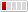0 Replies Latest reply on Feb 12, 2009 11:09 AM by Yogesh Bhardwaj

# [JBoss Rules/Drools] - Mathematical formula implementationHello all,

I could not find a specific forum for Drools, so posting it here, please point me to it if there is one. I need an opinion from the Drools users/developers for a business problem.
We have a requirement in which the end users would create mathematical "formulae" through a UI. So in a single transaction, a user could create a formula, apply it on the transactional data, and save the formula for future use. The operands in a formula would be attributes of certain objects, and the operators could potentially go beyond just simple algebraic operators. An example could look like this:

```if (object.country == "USA")
then output = (object.normalRate * (@someConstant + #someUserInput)) / object.quantity
else output = (object.exciseRate * @someConstant) / object.quantity
```

The "decision-making" behavior of the formula inclines me towards using some rules engine like JBoss Rules/Drools. Does anyone here know if JBoss Rules or any other rules engine would fit for a requirement like this, where rules could be created dynamically through a UI and then saved (file or database) or users could select a rule among several available ones?

I would really appreciate any feedback.

Thanks,
Yogesh